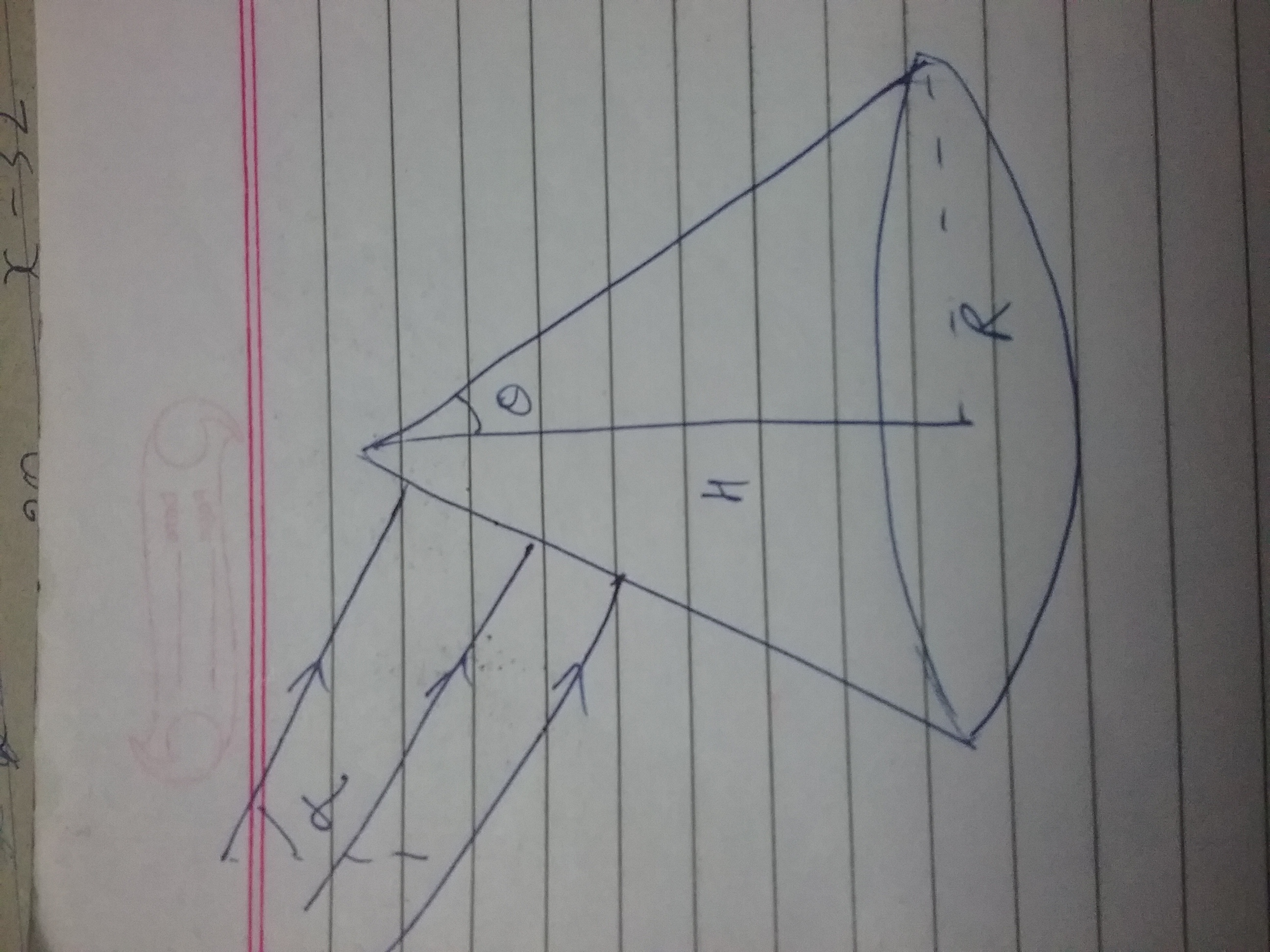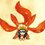A light of intensity $I$ is incident at an angle $\alpha$ with the vertical on a cone with semivertical angle $\theta<\alpha$, height $H$ and radius $R$

▪ Supposing that the cone is perflectly reflecting i.e. does not absorb or transmit

▪ Supposing that cone is perfectly absorbing i.e. does not reflect or transmit

Find the radiation force on cone in both cases.Note by Prince Loomba
4 years, 9 months ago

This discussion board is a place to discuss our Daily Challenges and the math and science related to those challenges. Explanations are more than just a solution — they should explain the steps and thinking strategies that you used to obtain the solution. Comments should further the discussion of math and science.

When posting on Brilliant:

• Use the emojis to react to an explanation, whether you're congratulating a job well done , or just really confused .
• Ask specific questions about the challenge or the steps in somebody's explanation. Well-posed questions can add a lot to the discussion, but posting "I don't understand!" doesn't help anyone.
• Try to contribute something new to the discussion, whether it is an extension, generalization or other idea related to the challenge.

MarkdownAppears as
*italics* or _italics_ italics
**bold** or __bold__ bold
- bulleted- list
• bulleted
• list
1. numbered2. list
1. numbered
2. list
Note: you must add a full line of space before and after lists for them to show up correctly
paragraph 1paragraph 2

paragraph 1

paragraph 2

[example link](https://brilliant.org)example link
> This is a quote
This is a quote
    # I indented these lines
# 4 spaces, and now they show
# up as a code block.

print "hello world"
# I indented these lines
# 4 spaces, and now they show
# up as a code block.

print "hello world"
MathAppears as
Remember to wrap math in $$ ... $$ or $ ... $ to ensure proper formatting.
2 \times 3 $2 \times 3$
2^{34} $2^{34}$
a_{i-1} $a_{i-1}$
\frac{2}{3} $\frac{2}{3}$
\sqrt{2} $\sqrt{2}$
\sum_{i=1}^3 $\sum_{i=1}^3$
\sin \theta $\sin \theta$
\boxed{123} $\boxed{123}$

Sort by:

For the 2nd case, I have used projection area. It is semicircle and a semi ellipse,

So we can write area=$\frac {\pi R^{2}}{2}+\frac {\pi RH}{2}$

And then use $IA/c$

Is this approach right?

And I have no idea for the first case

- 4 years, 9 months ago

for the first case the force will become twice of what u have found in the second case .change in momentum will be 2h/lambda .

And if it was a case where here was reflection and absorbtion .then u calculate the force in each case and multiply it with the coefficient of absorbption and reflection respectively (which would be specified in the question )(also to note that a+r=1 where a and r are the coefficients of absorbtion and reflection respectively )

- 4 years, 8 months ago

Exactly twice, are you sure? And I havent really found a proof for other half to be ellipse, can you find?

- 4 years, 8 months ago

ill try proving it .

- 4 years, 8 months ago

Thanks

- 4 years, 8 months ago

how did u conclude that it would be an area of ellipse only in the first part ?

- 4 years, 8 months ago

I havent. For second I said its half ellipse half corcle, with no proof

- 4 years, 8 months ago